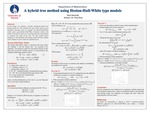## Stander Symposium Posters

#### Description

In this poster, we consider a recently introduced hybrid tree pricing model. We consider the case where there are two stochastic processes and we consider the Heston-Hull-White model, and a generalized Hull-White stochastic interest rate model. We build two trees (X, V) and then take the cross product to construct the mesh of the tree. We then predict prices analogous to the binomial asset pricing model. To validate the model we compare the results to a simulation using the Monte Carlo method. We shall consider the case where there is zero correlation between the two stochastic processes and the case where there is nonzero correlation between the two stochastic processes.

4-24-2019

Paul W Eloe

Mathematics

#### Keywords

Stander Symposium poster